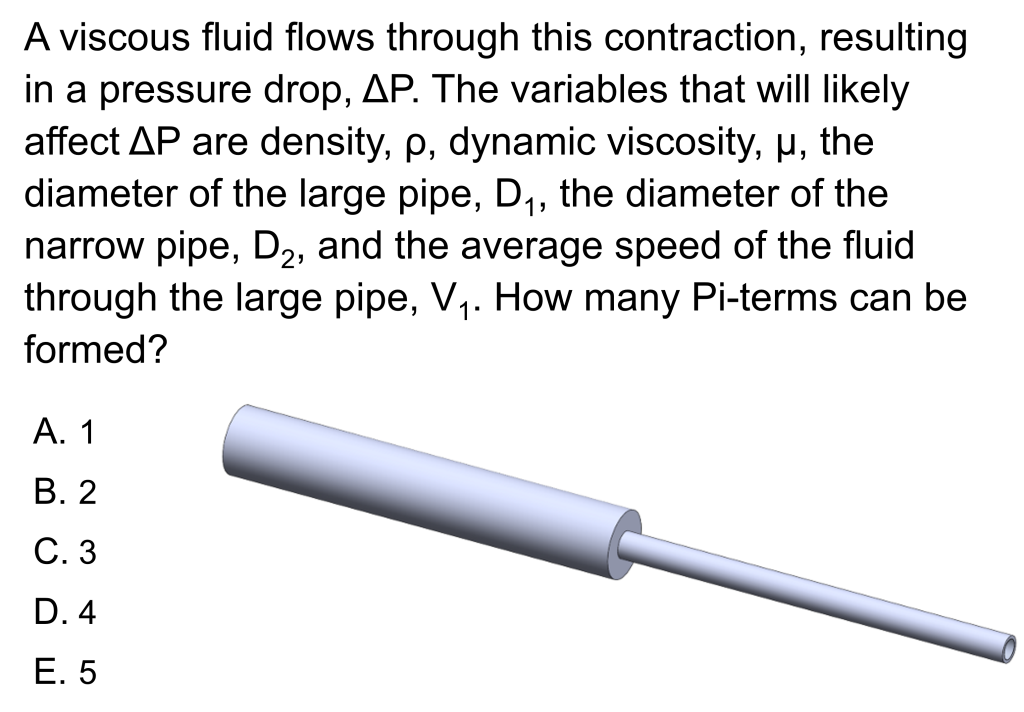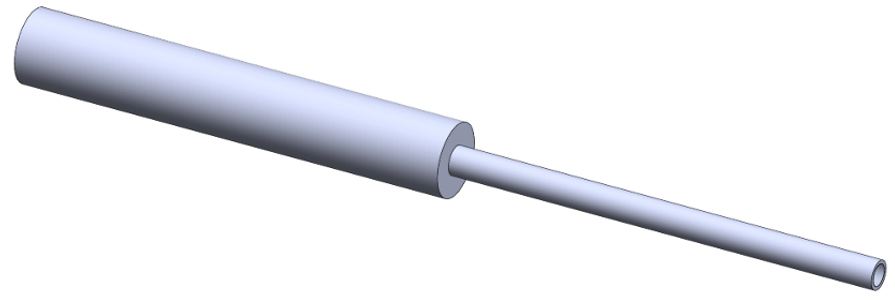LearnChemE

#### Dimensional Analysis, Pi Terms, and Dimensionless Numbers: ConcepTest and Example Problem

Try to answer this ConcepTest and solve the example problem before using this module. Studies show that trying to answer the questions before studying material improves learning and retention. We suggest that you write down the reasons for your answers. By the end of this module, you should be able to answer these on your own. Answers will be given at the end of this module.##### Example Problem 1

A viscous fluid flows through this contraction. You need to estimate the pressure drop, ΔP, that is caused by the contraction. Bernoulli’s equation cannot be used because the fluid is quite viscous, and the N-S equations cannot be simplified enough to integrate. Thus, you’ll need experimental data to find ΔP. Determine all dimensionless groups (Π-terms) needed to analyze this situation with experiments. The variables that will likely affect ΔP are density, ρ, dynamic viscosity, μ, the diameter of the large pipe, D1, the diameter of the narrow pipe, D2, and the average speed of the fluid through the large pipe, V1.